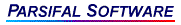## evaluateExpression: A Function to Evaluate Arithmetic ExpressionsevaluateExpression is a convenient utility for parsing and evaluating arithmetic expressions at runtime. You can use it, for example, to evaluate expressions read from data files or configuration files or expressions entered directly by users. evaluateExpression is provided as an example of the use of the AnaGram parser generator.

This example has been specially constructed so that you can easily adapt it to your specific requirements, even if you do not have a copy of AnaGram.

evaluateExpression may be freely copied and modified.

To use the evaluateExpression function in your program simply add EVALKERN.C and EVALWRAP.C (with headers EVALKERN.H and EVALDEFS.H) to your project and call the function as described below.

The evaluateExpression example consists of seven files:

• A syntax file, EVALKERN.SYN, which defines the parser itself.
• The c file EVALKERN.C, which has been generated by AnaGram from the syntax file
• The h file EVALKERN.H, similarly generated by AnaGram
• A wrapper module, EVALWRAP.C, which implements support functions and the evaluateExpression interface function.
• A header file, EVALDEFS.H, which provides global declarations.
• A demo program, EVALDEMO.C, which illustrates the use of evaluateExpression.
• A test file, TEST, which contains a self-commented, sample expression string with a variety of expressions.### int evaluateExpression(char *expressionString);

evaluateExpression is defined in the wrapper module EVALWRAP.C. The example has been set up with a wrapper module to allow you more flexibility in case you do not have a copy of AnaGram. You can modify the wrapper module to suit your needs. evaluateExpression calls the parser function, which is named evalKernel(). The parser file, which has been produced by AnaGram from the specs in EVALKERN.SYN, is EVALKERN.C.

EVALWRAP.C also has definitions of the functions called by the parser, which are pushChar, locateVariable, pushArg, callFunction, and checkZero. You can modify these functions as necessary.

Finally, EVALDEMO.C is provided as an example of a way you might use evaluateExpression. It parses an input file containing expressions and comments, evaluates the expressions, and outputs the (final) value of each variable in the symbol table. Note that the symbol table is alphabetically sorted. Since the output is in a form which is itself readable by the parser, EVALDEMO could be easily modified to accept previous output as initialization of the symbol table.

### Expression Syntax

expressionString is a null terminated text string. It may contain any number of expressions separated by commas or semicolons. White space may be used freely, including both C and C++ style comments. Function calls may have any number of arguments.

The expression syntax is borrowed from C but with the addition of the FORTRAN exponentiation operator (**).

Examples:

```   y1 = 21, LongVariableName = 12.5;  // simple assignment
y1 += (1/(c=.05)) ;                // add to memory, with parenthesized assignment
testx = x >=z ? z : 1 ;            /* conditional expression */
u = sin(x), v = cos(y);            // Standard math functions
```
EVALDEMO will accept the above lines as input either individually or together. See the TEST file for further expression examples.

Operations:

The cast, increment, and decrement operators are not implemented, nor are the following operations that are defined in C only for integers:

```    Bitwise logical operators:    &, |, ^, ~, &=, |=, ^=
Remainder operators:          %, %=
Shift operators:              <<, >>, >>=, <<=
```

The supported operations are:

```    Assignment operators:         =   +=   -=   *=   /=
Conditional expressions:      ?  :
Logical operators:            !  &&  ||
Comparison operators:         ==  !=  <  <=  >  >=
Binary arithmetic operators:  +  -  *  /
Exponentiation:               **
Unary arithmetic operators:   +  -
Parentheses                   (  )
Function calls                ...( ... )
```

All arithmetic is double precision floating point.

### Character Stack

The parser presumes the existence of a character stack. The parser pushes characters onto the stack by calling an externally defined function,

```    void pushChar(int c);
```

To pass a character string to an external function (locateVariable, callFunction), the parser pushes the characters onto the stack and then provides the function with the length of the string. It presumes the function will pop the characters from the stack.

### Variables

Variable names follow conventional C rules. The parser imposes no limits on the length of variable names. There is no built in symbol recognition logic in the parser module itself. To identify the location where the value of a variable is stored, the parser calls

```    double *locateVariable(int nameLength);
```

nameLength is the length of the variable name on the character stack. locateVariable pops the name from the stack and returns a pointer to the value of the variable.

N.B. If the name does not yet appear in the symbol table, the sample implementation of locateVariable adds it to the table and initializes its value to zero.

The implementation of locateVariable provided in the wrapper module is intended only as an example. Users with specific requirements might wish to modify it or replace it with something more suitable to their needs.

### Function Calls

Function names are not built into the parser, so that the user may incorporate the functions he needs. When the parser encounters a function call, it evaluates the arguments and calls an externally defined pushArg function to save them on a stack, then invokes a callback function:

```    double callFunction(int nameLength, int argCount);
```

to identify the function and evaluate it. The name of the function, containing nameLength characters, is on the character stack, argCount specifies the number of arguments on the the argument stack. callFunction must remove the function name from the character stack and the arguments from the argument stack.

The implementation of callFunction found in the wrapper module provides only for the standard C library math functions. Other functions can be added easily.

### Return Value

evaluateExpression returns 0 if there are no errors; otherwise it returns a non-zero value.

AnaGram parser generator - examples
Main page - evaluateExpression